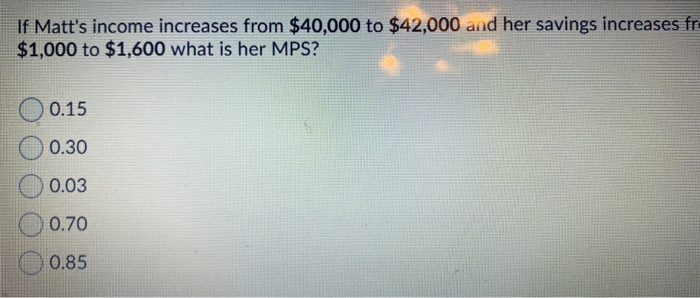# If Matt's income increases from $40,000 to$42,000 and her savings increases fr. $1,000 to$1,600...

###### Question:If Matt's income increases from $40,000 to$42,000 and her savings increases fr. $1,000 to$1,600 what is her MPS? O 0.15 0.30 0.03 0.70 0.85

##### Find the equation and length of perpendicular from a point P(-3,0,-2) to the line (x-2)/2=(y-2)/(-2)=(z-1)/1?
Find the equation and length of perpendicular from a point P(-3,0,-2) to the line (x-2)/2=(y-2)/(-2)=(z-1)/1?...